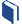# Making Deviation Standard

###Resources for this lesson:

> Glossary> Calculator Resources> Teacher Resources: Instructional NotesWhat is the difference between the mean absolute deviation and standard deviation? For the data on the years the presidents served, the mean was 5.071 years with a mean absolute deviation of 2.033 and a standard deviation of 2.445.Which is better to use? Mean absolute deviation is actually more descriptive when describing the distribution of data. However, standard deviation is more widely accepted and used by statisticians. As you work more with mean absolute deviation and standard deviation, you will notice that the values tend to be approximately equal for smaller data sets and very much unequal for larger data sets

President George W. Bush

The standard deviation is not necessarily better or worse than the mean absolute deviation; it is just more commonly used. You will find, as in the lessons that follow, graphing calculators and computer software favor the standard deviation. Again, this is due to the fact that statisticians and mathematicians have come to accept standard deviation over mean absolute deviation.